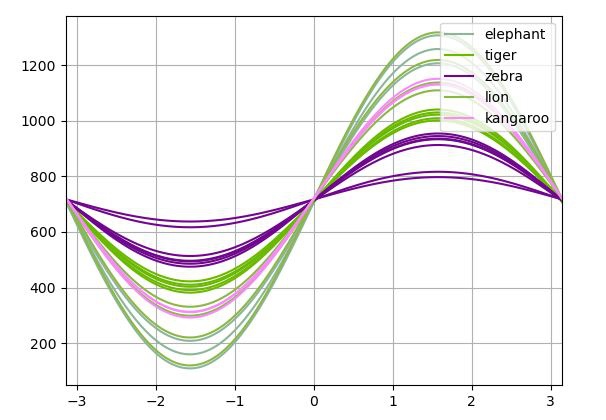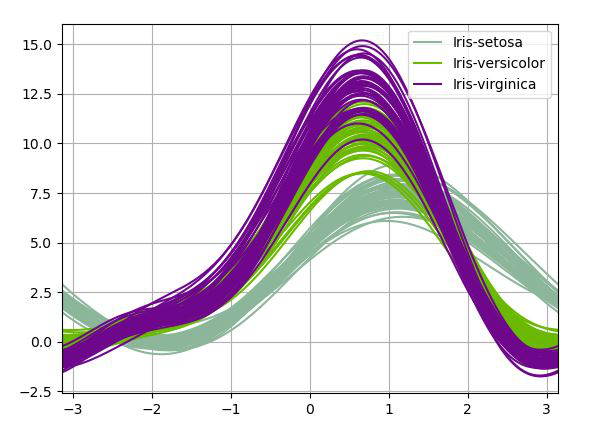# How to plot Andrews curves using Pandas in Python?

• Last Updated : 01 Aug, 2020

Andrews curves are used for visualizing high-dimensional data by mapping each observation onto a function. It preserves means, distance, and variances. It is given by formula:

T(n) = x_1/sqrt(2) + x_2 sin(n) + x_3 cos(n) + x_4 sin(2n) + x_5 cos(2n) + …

Plotting Andrews curves on a graph can be done using the andrews_curves() method of the plotting module. This function generates a matplotlib plot of Andrews curves, for visualising clusters of multivariate data.

Syntax: andrews_curves(frame, class_column, ax=None, samples=200, color=None, colormap=None, **kwargs)

Parameters:

• frame: It is the data to be plotted.
• class_column: This is the name of the column containing class names.
• ax: This parameter is a matplotlib axes object. Its default value is None.
• samples: This parameter is the number of points to plot in each curve.
• color: This parameter is an optional parameter and it is the list or tuple of colors to use for the different classes.
• colormap: This parameter is the string/matplotlib colormap object. Its default value is None.

Returns: This function returns an object of class matplotlip.axis.Axes

Example 1: In the following example, A data frame is made from the CSV file and the data frame is used to plot andrews_curves. The used CSV file is here.

## Python3

 `# importing various package``import` `pandas as pd``import` `numpy as np``import` `matplotlib.pyplot as plt`` ` `# making data frame from csv file``df ``=` `pd.read_csv(``    ``'C:\\Users\\digital india\\Desktop\\pand.csv'``)`` ` `# Creating Andrews curves``x ``=` `pd.plotting.andrews_curves(df, ``'animal'``)`` ` `# ploting the Curve``x.plot()`` ` `# Display``plt.show()`

Output:Example 2:

## Python3

 `# importing various package``import` `pandas as pd``import` `numpy as np``import` `matplotlib.pyplot as plt`` ` `# making data frame from csv file``df ``=` `pd.read_csv(``    ``'https://raw.github.com/pandas-dev/'``    ``'pandas/master/pandas/tests/io/data/csv/iris.csv'``)`` ` `# Creating Andrews curves``x ``=` `pd.plotting.andrews_curves(df, ``'Name'``)`` ` `# ploting the Curve``x.plot()`` ` `# Display``plt.show()`

Output:My Personal Notes arrow_drop_up